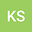loading page

Numerical Simulation of the Fractal Fractional Reaction Diffusion Equations with General Nonlinear
•••Khaled M Saad
Department of Mathematics, Faculty of Applied Science, Taiz University, Department of Mathematics, College of Sciences and Arts, Najran University
Manal Alqhtani
Department of Mathematics, College of Sciences and Arts, Najran University

## Abstract

In this paper we utilize the new operators using three different kernels,namely, power law, exponential decay, and the generalized Mittag-Leffler function based on the fractal-fractional differentiation. These constructed operators have two parameters: the first is considered as fractional order and the second as fractal dimension. We studied the effect of the fractal-fractional derivative order as well as the nonlinear term order 1 < q ≤ 2 on the behavior of the numerical solutions of the fractal-fractional reaction diffusion equations (FFRDE). The iterative approximations are constructed by applying the theory of fractional calculus with the help of Lagrange polynomial functions. In the case of β = k = 1 , all the numerical solutions based on power kernel, exponential kernel, and the generalized Mittag-Leffler kernel are very close to each other and so one of the kernel was compared with numerical methods like finite difference methods (FDM) and an excellent agreement obtained. All calculations in this paper were done using the mathematica package.